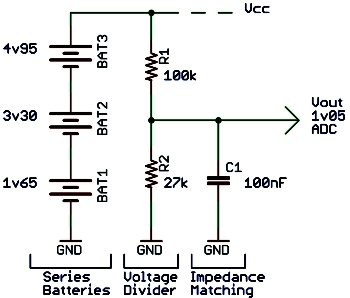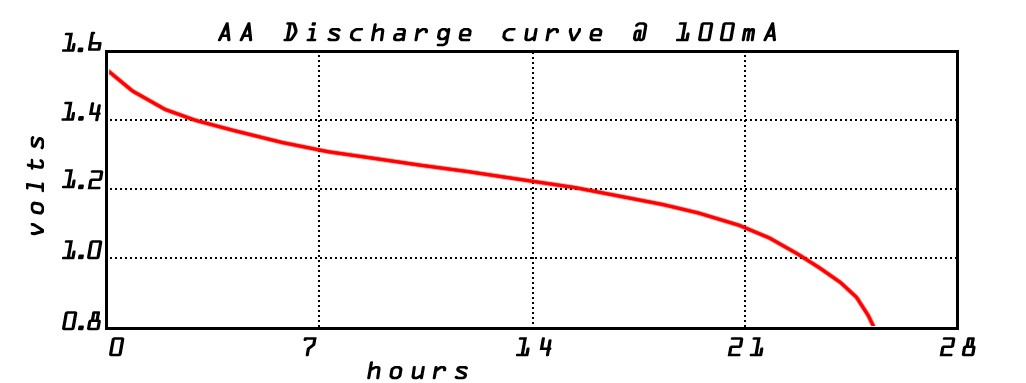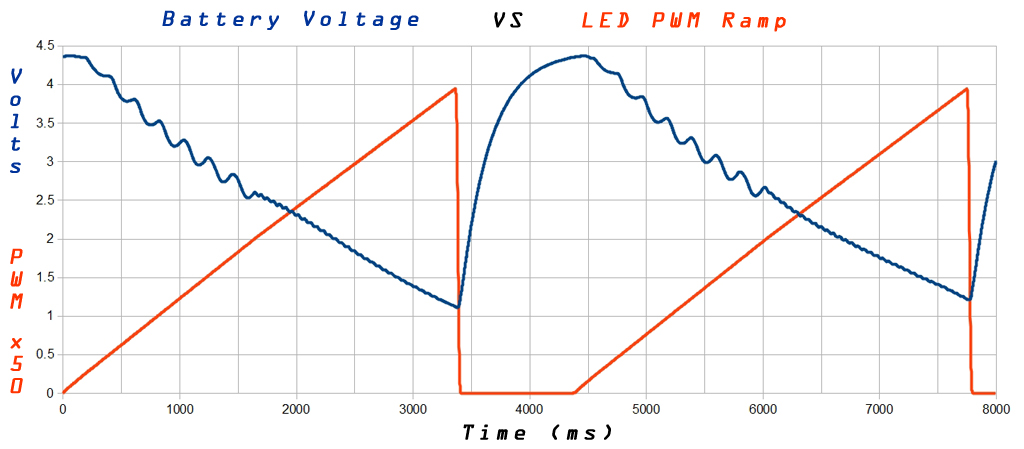# ADC Battery Voltage: Divide, Match and Measure…While the stock Arduino isn’t particularly well geared to running from a battery source, there are times it will be useful to know the status of our battery voltage.

Using the circuit on the left as well as a bit of math, we will discover how to accurately measure the battery level.  But first a bit of preliminary information about batteries to get us started…

Alkaline Voltage Characteristics

All battery chemistries have differing voltage characteristics.  For the purpose of this article, we will be considering standard alkaline batteries where the unloaded voltage can be as high as 1.65 volts per cell.  Utilizing three in series gives us a maximum combined output of 4.95 volts.  On the other side of the spectrum, when the unloaded cell voltage drops below 1.0 volt, more than 85% of the capacity has been relinquished.  For this reason we are most interested in the range from 3.0 to 4.95 volts or the per cell voltage range of 1.0 to 1.65 volts as can be seen on the discharge chart below:Analog to Digital Converter Setup

The Arduino and most current microcontrollers have a built in, easy to use ADC.  To accurately measure a voltage using the ADC, we first need to specify a reference for comparative purposes.  As shown below, this is done in the Arduino with the analogReference(type) command.  The available choices depend on your Arduino, we will be using the internal 1.1 volt reference,  other reference choices can be found here.  It’s a very good idea to bypass the Aref pin with a 100nF capacitor (not shown) if not already done. Here’s the setup code:

`analogReference(INTERNAL);`

Enter the Voltage Divider

As you might have guessed from the ADC setup, measuring the battery voltage is actually a comparison between the voltage on the analog pin and the 1.1 volt reference.  The reference voltage is the maximum and for this reason we don’t want to put any voltage higher than 1.1 volts on the analog input pin.  This is where the voltage divider comes in,  it scales the 3.0 – 4.95 v battery voltage to a lower range of 0.64 – 1.05 volts.  The math to calculate this uses the battery voltage and resistor values from the schematic at the top.  It can also be useful to express this equation in terms of R1 or R2 when selecting resistors:

```Vout = (Battery Voltage x R2) / (R1 + R2)
R1 = ((R2 x Battery Voltage) / Vout) - R2
R2 = R1 / ((Battery Voltage / Vout) - 1)```

Impedance Matching
& Power Consumption
While often overlooked, the most common source of ADC inaccuracy is from an impedance mismatch.  Simply put, the Arduino’s ADC input is expecting to see 10k ohms or less of resistance.  When there is more than 10k of input resistance, the Arduino’s own resistance starts to effect the measurement.  The combination of 27k and 100k resistors selected puts us well over the expected 10k impedance range.  We can lower our resistances but this will cause a larger power dissipation (P = V*V/R), not a great option for long battery life.  The easiest solution for slow changing signals is to add a 100nF ceramic capacitor (C1) to ground which will hold the voltage level while the ADC takes a measurement.

Taking a Measurement & Resolution that Counts

Returning to our measurement, this comparison produces a value out of the maximum resolution count, default being 10 bits or 1024 counts. Once we get our count we then work the math in reverse to determine what the battery voltage is.  Our measurement is taken with the command:

`count = analogRead(analogPin);`

The math to calculate the battery voltage depends on the full range (1024), the reference voltage as well as the ratio of the voltage divider resistances, first we figure out what Vout was at the time of measurement.  Then we use Vout to calculate the battery voltage, with the help of our resistive divider:

```count = (Vout x maximum count) / Reference voltage
Vout = (count / maximum count) x Reference voltage
Battery voltage = Vout x ( R1 + R2) / R2
Battery v = (count x ref voltage x (R1 + R2))/(R2 x max count)```

Putting It All Together

Making sense of it all, we have a final piece of code with a bit of massaging to improve our measurement quality.  Ideally we want to divide as little as possible as division leads to loss of resolution, hence we perform a composite rolling average and push the division to the end:

```count = analogRead(analogPin);

Rolling = Rolling - ( Rolling /8 );
Rolling = Rolling + count;

BatteryVoltage = ((Rolling * 1397) / 276480) /8;```

Finally in application, we have a pretty chart of the battery voltage dipping as a high power LED follows an incrementally increasing PWM duty cycle.  The LED is drawing up to 1.20 Amps through a switch mode boost converter while the battery supply dips to about 1.15 volts.  The supply droop is a result of the battery’s internal resistance, about 0.2 ohms per cell:### 7 Responses to “ADC Battery Voltage: Divide, Match and Measure…”

•Very well written article and you are doing a great job on your site! But I’m curious…why bother with the voltage divider? Why not just use 5.0V directly from the batteries as the reference voltage and take that as the input into an analog pin on the Arduino? What’s the downside of doing it that way?

•As you suggest, using the 5v from the battery as the reference, and measuring based on this reference will not work. The reference must be constant, otherwise, as the battery voltage falls so does the reference, as well as the measured voltage and the ADC will always read 1023.

There are cases in which you want to use the source voltage as reference, as when measuring a resistive bridge, but not when measuring the battery.

•could you please explain how you calculate the impedance? Say, I need to measure voltage in the fuel gauge circuit, which is +12v -> gauge coil (60 ohm) -> variable resistor (Fuel sending unit, 20-300Ohm) -> Ground.
I need to measure voltage between the gauge coil and the sending unit. What’s the impedance of this circuit?

•Serjio,

Michael

•This is a big help because I have an panStamp (Arduino Mini Pro 3.3 v + RF) that I want to power with a CR123 battery and I want to measure the battery voltage so I know when it’s low. I want to make sure I understand how the 100nF cap is working. When the analog input is not being read, it’s not drawing any current, so the voltage divider circuit can charge up the capacitor. Then when the Arduino reads the analog pin, the Arduino is getting the electricity from the capacitor, so the high impedance of R1 and R2 don’t come into play. I guess you could use even bigger resistors as long as the capacitor had time to charge up between readings. Is that right?

Also, what do you mean by “bypass the Aref pin with a 100nF capacitor”? And why is this a good idea?

•Hi Scott216,

Your understanding of the cap on the divider is absolutely correct, great explanation.
As for the bypassing the Aref pin, the datasheet indicates it’s for improved noise immunity.
It’s just a cap to ground and I’d guess it also helps impedance match the Aref source.
Some Arduinos already have it included, it will be on the schematic if it’s there…

Michael

•Hello i not understand your post…
can you explain me what are :